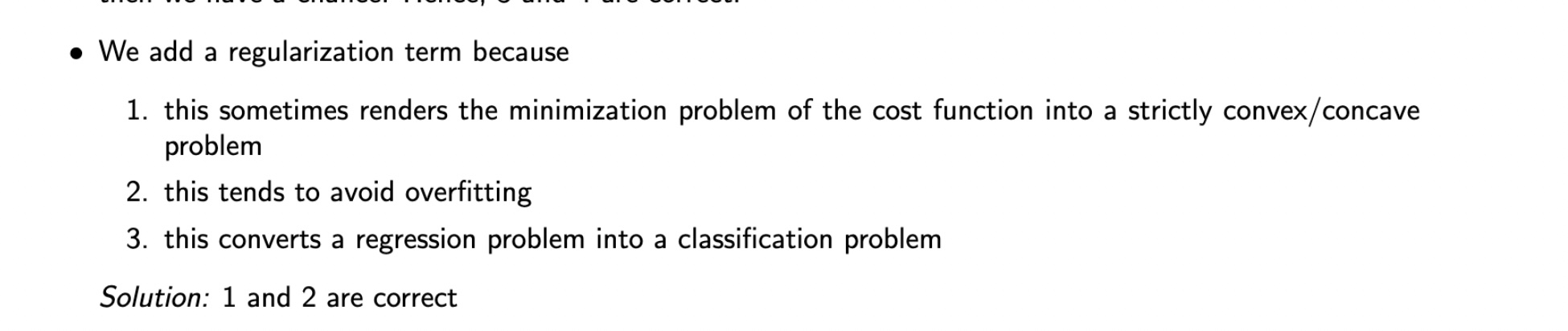### Mock 2018 QCM Regularization term

Hello,
Can you give me an example of an objective where we had a regularization term in order to render the minimization problem strictly convex/concave? :/ I am not convinced.Take linear regression for example, the hessian is given by $$XX^\top /N$$ for $$X\in \mathcal{R}^{d\times N}$$.
If this matrix is not full rank then the problem is not strictly convex and in particular we can't invert the matrix $$XX^\top$$, but with ridge regression we add a $$\lambda$$ to all directions and thus ensure that the smallest eigenvalue of the hessian is at least this $$\lambda$$, thus we have made the problem strictly convex.